Search

Matrix Row Operations (page 1 of 2)"Operations" is mathematician-ese for "procedures". The four "basic operations" on numbers are addition, subtraction, multiplication, and division. For matrices, there are three basic row operations; that is, there are three procedures that you can do with the rows of a matrix.

The first operation is row-switching. For instance, given the matrix: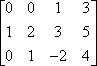...you can switch the rows around to put the matrix into a nicer row arrangement, like this: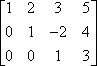Row-switching is often indicated by drawing arrows, like this: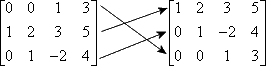When switching rows around, be careful to copy the entries correctly.

The second operation is row multiplication. For instance, given the following matrix: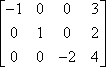...you can multiply the first row by 1 to get a positive leading value in the first row: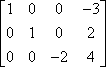This row multiplication is often indicated by using an arrow with multiplication listed on top of it, like this: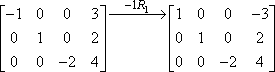The "1R1" indicates the actual operation. The "1" says that we multiplied by negative one; the "R1" says that we were working with the first row. Note that the second and third rows were copied down, unchanged, into the second matrix. The multiplication only applied to the first row, so the entries for the other two rows were just carried along unchanged.

You can multiply by anything you like. For instance, to get a leading 1 in the third row of the previous matrix, you can multiply the third row by a negative one-half: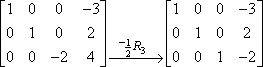Since you weren't doing anything with the first and second rows, those entries were just copied over unchanged into the new matrix.

You can do more than one row multiplication within the same step, so you could have done the two above steps in just one step, like this:It is a good idea to use some form of notation (such as the arrows and subscripts above) so you can keep track of your work. Matrices are very messy, especially if you're doing them by hand, and notes can make it easier to check your work later. It'll also impress your teacher.

The last row operation is row addition. Row addition is similar to the "addition" method for solving systems of linear equations. Suppose you have the following system of equations:

x + 3y = 1
x + y = 3

You could start solving this system by adding down the columns to get 4y = 4: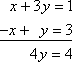You can do something similar with matrices.For instance, given the following matrix: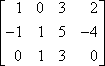...you can "reduce" (get more leading zeroes in) the second row by adding the first row to it (the general goal with matrices at this stage being to get a "1"  or "0's" and then a "1"  at the beginning of each matrix row). When you were reducing the two-equation linear system by adding, you drew an "equals" bar across the bottom and added down. When you are using addition on a matrix, you'll need to grab some scratch paper, because you don't want to try to do the work inside the matrix. So add the two rows on your scratch paper:

 Scratch work  don't hand this in!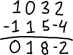This is your new second row; you will write it in place of the old second row. The result will look like this: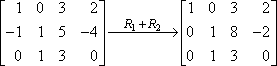In this case, the "R1 + R2" on the arrow means "I added row one to row two, and this is the result I got". Since row one didn't actually change, and since we didn't do anything with row three, these rows get copied into the new matrix unchanged.